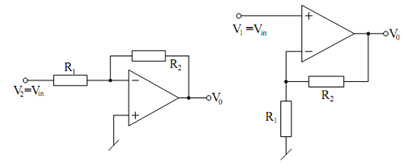## Inverting Amplifier Assignment Help

Assignment Help: >> Basic Analog Circuits using Ideal Op-amps - Inverting Amplifier

Inverting Amplifier:

When V1 = 0, V2 = Vin, then circuit reduces to the one illustrated in Figure (a) with

V0=- (R2/ R2)Vin

This circuit is known as an inverting amplifier. Even though the inverting input terminal is not connected to ground, thus far the infinite-gain and negative feedback force the voltage appearing on this terminal to be ground potential. Hence it is called a 'Virtual ground'. Note that circuit ideally has zero output impedance but input impedance is equal to R1 due to the virtual ground at the inverting input terminal of the op-amp.(a)                                                   (b)

Figure: (a) Inverting Amplifier; and (b) Non-inverting Amplifier

Case 2

If V2 = 0, V1 = Vin, then the circuit takes the form illustrated in Figure (b) with

V0  =  (1 +( R2 /R1)  ) V in

This circuit is called a non-inverting amplifier. Note down that this circuit does have ideally infinite input impedance.